# DAV Class 8 Maths Chapter 4 Brain Teasers Solutions

The DAV Class 8 Maths Book Solutions and DAV Class 8 Maths Chapter 4 Brain Teasers Solutions of Direct and Inverse Variation offer comprehensive answers to textbook questions.

## DAV Class 8 Maths Ch 4 Brain Teasers Solutions

Question 1A.
Tick (✓) the correct option.

(i) Both x and y are in direct proportion, then $$\frac{1}{x}$$ and $$\frac{1}{y}$$ are
(a) in direct proportion
(b) in inverse proportion
(c) neither in direct nor in inverse proportion
(d) sometimes in direct and sometimes in inverse proportion
Solution:
(a) in direct proportion

(ii) If two quantities x and y vary inversely with each other, then
(a) $$\frac{x}{y}$$ remains constant
(b) (x – y) remains constant
(c) (x + y) remains constant
(d) (xy) remains constant
Solution:
(d) (xy) remains constant

(iii) Both p and q vary directly with each other. When p is 10 and q is 15, which of the following is not a possible pair of corresponding values of p and q?
(a) 15 and 20
(b) 20 and 30
(c) 2 and 3
(d) 5 and 7.5
Solution:
(a) 15 and 20

(iv) If x and y vary directly with each other and x variation is 3?
(a) 21
(b) $$\frac{1}{8}$$
(c) 8
(d) 27
Solution:
(c) 8
$$\frac{x}{y}$$ = 3
⇒ $$\frac{24}{y}$$ = 3
⇒ y = $$\frac{24}{3}$$
⇒ y = 8.

(v) Which of the quantities vary inversely with each other?
(a) Distance travelled and cab fare.
(b) Area of a land and its cost.
(c) Number of workers and amount of work done.
(d) Number of workers and time taken to finish a job.
Solution:
(d) Number of workers and time taken to finish a job.Question 1B.

(i) l varies directly as m and l is equal to 5, when m = $$\frac{2}{3}$$. Find l when m = $$\frac{16}{3}$$.
(ii) A bowler throws a cricket ball at a speed of 36 km/hr. How long does this ball take to travel a distance of 20 metres to reach the batsman?
(iii) Sweets from a packet were distributed among 50 children and each of them received four sweets. If it is distributed among 40 children, how many sweets will each child get?
(iv) If y is directly proportional to $$\frac{1}{x}$$ and x = 2 when y = 20, what is the value of x when y = 1.25?
(v) If l is inversely proportional to √m and l = 6 when m = 4. What is the value of m when l = 4?
Solution:
(i) Let the required value of l be x.As this is a case of direct variation.

∴ $$\frac{5}{2 / 3}=\frac{x}{16 / 3}$$
⇒ $$\frac{2}{3} x=5 \times \frac{16}{3}$$
⇒ x = 5 × $$\frac{16}{3}$$ × $$\frac{3}{2}$$ = 40

(ii) Speed = 36 km/hr = $$\frac{36 \times 1000}{60 \times 60}$$ m/s
= 10 m/s
Time taken = $$\frac{\text { Distance }}{\text { Speed }}=\frac{20}{10}$$
= 2 seconds

(iii) Let the number of sweets be x.It is a case of indirect variation.
Thus, 50 × 4 = 40 × x
∴ x = $$\frac{50 \times 4}{40}$$
= 5 sweets

(iv) Let the required value of x be a.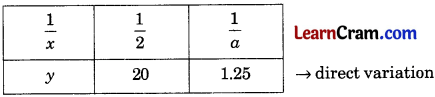It is a case of direct variation.
∴ $$\frac{\frac{1}{2}}{20}=\frac{\frac{1}{a}}{1.25}$$
⇒ $$\frac{1}{2}$$ × 1.25
= $$\frac{1}{a}$$ × 20
a = $$\frac{2 \times 20}{1.25}$$
= $$\frac{40}{1.25}$$ = 32.

(v) Let the required value of m be x.It is a case of indirect proportional.
As it is given that
l ∝ $$\frac{1}{\sqrt{m}}$$
⇒ l√m = k
Now, 6 × √4 = 4 × √x
√x = $$\frac{6 \times \sqrt{4}}{4}$$
x = $$\left(\frac{6 \times \sqrt{4}}{4}\right)^2$$
= $$\frac{36 \times 4}{4 \times 4}$$ = 9.Question 2.
Determine from the values of x and y given below whether they vary directly, inversely or in neither of these ways.

(i)(ii)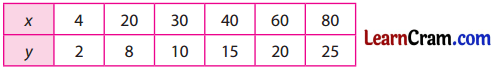(iii)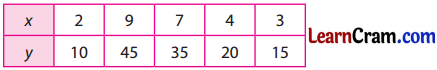Solution:
(i) $$\frac{x}{y}=\frac{12}{9} \neq \frac{3}{36} \neq \frac{27}{4} \neq \frac{6}{18} \neq \frac{2}{54}$$
So, they are not directly proportional.
For inversely proportional,
x1y1 = x2y2
So, 12 × 9 = 3 × 36
= 27 × 4
= 6 × 18
= 2 × 54
= 108
Hence, they vary inversely.

(ii) $$\frac{x}{y}=\frac{4}{2} \neq \frac{20}{8} \neq \frac{30}{10} \neq \frac{45}{15} \neq \frac{60}{20} \neq \frac{80}{25}$$
So, they do not vary directly.
For inversely proportional,
x1y1 = x2y2
So, 4 × 2 ≠ 20 × 8 ≠ 30 × 10 ≠ 40 × 15 ≠ 60 × 20 = 80 × 25
Hence, they are neither directly nor inversely proportional.

(iii) $$\frac{x}{y}=\frac{2}{10}=\frac{9}{45}=\frac{7}{35}=\frac{4}{20}=\frac{3}{15}=\frac{9}{45}$$
Hence, they vary directly.Question 3.
If x and y vary inversely and x = 25, when y = 3. Find y, when x = 15.
Solution:
Here x1 = 25, y1 = 3
x2 = 15 and y2 = y
If they vary inversely, then
x1y1 = x2y2
⇒ 25 × 3 = 15 × y
⇒ y = $$\frac{25 \times 3}{15}$$
⇒ y = 5.
Hence, the value of y is 5.

Question 4.
If x and y vary inversely and y = 45, find x when constant of variation = 9.
Solution:
If x andy vary inversely, then
x1y1 = x2y2 = K (constant variation)
∴ x × 45 = 9
⇒ x = $$\frac{9}{45}=\frac{1}{5}$$
Hence, the value of x is $$\frac{1}{5}$$.

Question 5.
Oranges cost 54 for 3 dozens in the super market. What is the cost of 8 oranges?
Solution:
Let the required cost be x.[∵ 3 dozen = 3 × 12 = 36]
As there is a direct variation,
$$\frac{36}{54}=\frac{8}{x}$$
⇒ 36 × x = 8 × 54
⇒ x = $$\frac{8 \times 54}{36}$$
⇒ x = 12
Hence, the required cost is ₹ 12.Question 6.
A car travels 60 km in 1 hr 30 min. How long will it take to cover a distance of 100 km at the same speed?
Solution:
Let the required time be t hours.[∵ 1 hr 30 min = 1.5 hours]
As there is a direct variation,
$$\frac{60}{1.5}=\frac{100}{t}$$
⇒ 60 × t = 100 × 1.5
⇒ t = $$\frac{100 \times 1.5}{60}$$
⇒ t = 2.5 hours = 2 hours 30 min.
Hence, the required time is 2 hours 30 min.

Question 7.
The extension of an elastic spring is found to vary directly with the weight suspended from it. If a weight of 75 kg produces an extension of 1.4 cm, calculate the weight that would produce an extension of 9.8 cm?
Solution:
Let the required weight be W kg.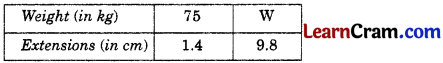As there is a direct variation,
$$\frac{75}{1.4}=\frac{W}{9.8}$$
⇒ 1.4 × W = 75 × 9.8
⇒ W = $$\frac{75 \times 9.8}{1.4}$$
⇒ W = 525 kg
Hence, the required weight is 525 kg.

Question 8.
In 25 days, the earth picks up 6 × 108 pounds of dust from the atmosphere. How much dust will it pick up in 15 days?
Solution:
Let the required dust to be pick up be x pounds.As there is direct variation,
$$\frac{6 \times 10^8}{25}=\frac{x}{15}$$
⇒ x × 25 = 15 × 6 × 108
⇒ x = $$\frac{15 \times 6 \times 10^8}{25}$$
⇒ x = 3.6 × 108
Hence, the required amount of dust in 15 days = 3.6 × 108 pounds.### DAV Class 8 Maths Chapter 4 HOTS

Question 1.
While driving his car at a speed of 50 km/hr, Ramit covers a distance from home to his office in 1 hour 30 minutes. One day he was 15 minutes late from his home. In order to reach office at time, what should be the speed of the car?
Solution:
Also, find the total distance covered by Ramit daily.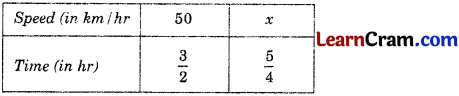It is a case of inverse variation.
∴ 50 × $$\frac{3}{2}$$ = x × $$\frac{5}{4}$$
⇒ x = 50 × $$\frac{3}{2} \times \frac{4}{5}$$
= 60 km/hr
Now, speed = 50 km/hr
and time = $$\frac{3}{2}$$ hr
distance covered = 50 × $$\frac{3}{2}$$ = 75 km
Total distance covered daily = 75 km + 75 km = 150 kmQuestion 1.
The cost of 8 mineral water bottles is ₹ 120. Find the cost of such 18 bottles?
Solution:
Let the required cost be ₹ x.As there is a direct variation,
$$\frac{8}{120}=\frac{18}{x}$$
⇒ 8 × x = 18 × 20
⇒ x = $$\frac{18 \times 120}{8}$$
⇒ x = 270
Hence, the number of required bottles are 270.

Question 2.
Ramesh deposited a sum of ₹ 12000 in a nationalised Bank at a certain rate of interest for 2 years and earns an interest of ₹ 900. How much interest would he earn for a deposit of ₹ 15000 for the same period and at the same rate of interest?
Solution:
Let the required amount of interest be ₹ x.As there is a direct variation,
$$\frac{12000}{900}=\frac{15000}{x}$$
⇒ 12000x = 15000 × 900
⇒ x = $$\frac{15000 \times 900}{12000}$$
⇒ x = 1125
Hence, the required amount of interest is ₹ 1125.Question 3.
In a picnic there is a provision for 400 students for 20 days. If 80 more students attend the picnic, for how many days will the provision be last?
Solution:
Let the number of days be x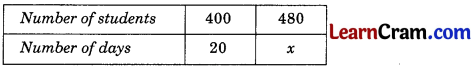[∵ Total number of students = 400 + 80 = 480]
As there is an inverse variation,
x1y1 = x2y2
⇒ 400 × 20 = 480 × x
⇒ x = $$\frac{400 \times 20}{480}$$
⇒ x = $$\frac{50}{3}$$ days = 16$$\frac{2}{3}$$ days
Hence, the required number of days = 16$$\frac{2}{3}$$.

Question 4.
An electric pole, 20 m high, casts a shadow of 15 m. Find the height of the tree that casts a shadow of 24 m under the similar conditions.
Solution:
Let the height of the tree required be x m.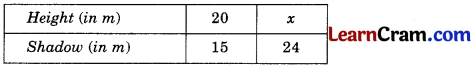As there is a direct variation,
∴ $$\frac{x_1}{y_1}=\frac{x_2}{y_2}$$
⇒ $$\frac{20}{15}=\frac{x}{24}$$
⇒ 15 × x = 20 × 24
⇒ x = $$\frac{20 \times 24}{15}$$
⇒ x = 32
Hence, the required height of tree is 32 m.Question 5.
A loaded truck travels 14 km in 25 minutes. If the speed of the truck remains the same, how far can it travel in 5 hours?
Let the required distance travelled be x km.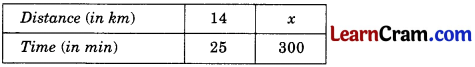[∵ 5 hours = 5 × 60 = 300 minutes]
$$\frac{x_1}{y_1}=\frac{x_2}{y_2}$$
⇒ $$\frac{14}{25}=\frac{x}{300}$$
⇒ 25 × x = 14 × 300
⇒ x = $$\frac{14 \times 300}{25}$$
⇒ x = 168.
Hence, the required distance is 168 km.

Question 6.
A factory requires 42 machines to produce a given number of articles in 63 days. How many machines would be required to produce the same number of articles in 54 days?
Solution:
Let the required number of machines be x.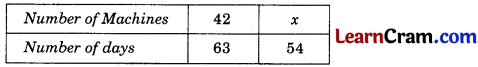[Total number of animals = 20 + 10 = 30]
As there is an inverse variation,
x1y1 = x2y2
⇒ 42 × 63 = x × 54
⇒ x = $$\frac{42 \times 63}{54}$$
⇒ x = 49
Hence, the required number of machines is 49.Question 7.
A farmer has enough food to feed 20 animals in his cattle for 6 days. How long would the food last if there were 10 more animals in his cattle?
Solution:[Total number of animals = 20 + 10 = 30]
As there is an inverse variation,
x1y1 = x2y2
⇒ 20 × 6 = 30 × x
⇒ x = $$\frac{20 \times 6}{30}$$
⇒ x = 4
Hence, the number of days required is 4 days.

Question 8.
A batch of bottles were packed in 25 boxes with 12 bottles in each box. If the same batch is packed using 20 bottles in each box, how many boxes would be filled?
Solution:
Let the required number of boxes be x.As there is an inverse variation,
x1y1 = x2y2
⇒ 25 × 12 = x × 20
⇒ x = $$\frac{25 \times 12}{20}$$
⇒ x = 15
Hence, the required number of boxes be 15.Question 9.
A car travels 60 km in 1 hr 30 min. How long will it take to cover a distance of 100 km at the same speed?
Solution:
Let the required time be x hours.$$\frac{60}{1.5}=\frac{100}{x}$$
⇒ 60 × x = 10 × 1.5
⇒ x = $$\frac{100 \times 1.5}{60}$$
⇒ x = $$\frac{5}{2}$$ = 2 hours 30 min.
Hence, the required time is 2 hours 30 min.

Question 10.
Ramesh can finish his work in 25 days, working 8 hrs a day. If he wants to finish the same work in 20 days, how many hours should he work in a day?
Solution:
Let the number of hours required be x.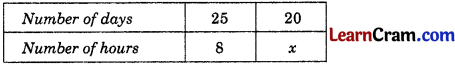As there is an inverse variation,
x1y1 = x2y2
⇒ 25 × 8 = 20 × x
⇒ x = $$\frac{25 \times 8}{20}$$
⇒ x = 10
Hence, the required time is 10 hours.Question 11.
A garrison of 120 men has provisions for 30 days. At the end of 5 days, 5 more men joined them. How many days can they sustain on the remaining provision?
Solution:
Let the number of days be x.[∵ Remaining days = 30 – 5 = 25]
[∵ Total men = 120 + 5 = 125]
As there is an inverse variation,
∴ x1y1 = x2y2
⇒ 120 × 25 = 125 × x
⇒ x = $$\frac{120 \times 25}{125}$$
⇒ x = 24
Hence, the required days are 24.